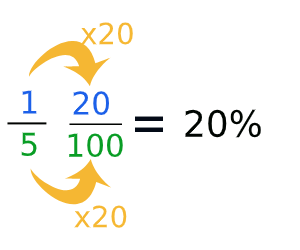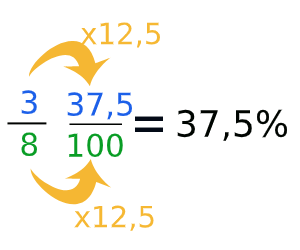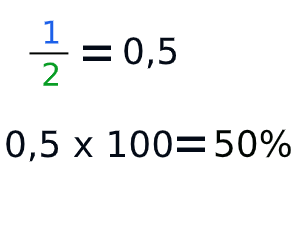# From Fractions to Percentage

## How do you change a fraction into a percentage?

If you want to change a fraction into a percentage, you need to multiply the fraction by 100. In these 3 examples we show you how you can change a fraction into a percentage. Or, in example 3, from a fraction to a decimal to a percentage. Before you start, it's good to know how a fraction works. The top number is the numerator, the bottom number is the denominator. These 2 numbers are necessary to go from a fraction to a percentage.

14 numeratordenominator

On this page you will find examples and exercices. For an extensive practice go to one of the 5-step plans.

### Example 1

In the first example we'll change the fraction 15 into a percentage.The goal of this method is to make the bottom number, the denominator, 100. In this case we need to change 5 into 100. A smart way to do this is to divide 100 by 5. That's 20. Then you know you have to do 5 x 20 to get to 100. when you multiply the denominator, you also need to multiply the numerator. So here we also multiply 1 by 20. When you've done all of that, you have the final fraction. In the final fraction, the numerator is the same as the percentage, 20%.

### Exercise 1:

Number of questions:

Time per question::

Tip: use tab to go to the next field

### Example 2

In the first example we'll change the fraction 38 into a percentage.Just like the previous example, we'll try to make the denominator 100. 100 divided by 8 is 12.5. Now we know that the denominator and the numerator need to be multiplied by 12.5. In the final fraction the numerator is 37.5 and now we know the percentage.

### Example 3You can also change a fraction into a percentage by calculating the decimal number. When you have the decimal number, you need to multiply that by 100, the answer is your percentage. This is a quick way, especially on the calculator. Numerator divided by denominator multiplied by 100.